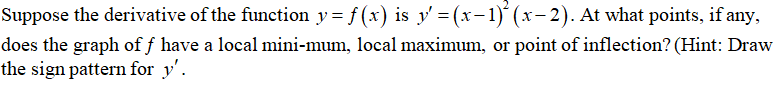# Suppose the derivative of the function y = ƒ(x) is y′ = (x - 1)2(x - 2). At what points, if any, does the graph of ƒ have a local mini-mum, local maximum, or point of inflection? (Hint: Draw the sign pattern for y′.

Question
2 views

Suppose the derivative of the function y = ƒ(x) is y′ = (x - 1)2(x - 2). At what points, if any, does the graph of ƒ have a local mini-mum, local maximum, or point of inflection? (Hint: Draw the sign pattern for y′.

check_circle

Step 1...

### Want to see the full answer?

See Solution

#### Want to see this answer and more?

Solutions are written by subject experts who are available 24/7. Questions are typically answered within 1 hour.*

See Solution
*Response times may vary by subject and question.
Tagged in